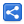# Getting Started with GPU programming using Aparapi Framework

[Updated: Feb 23, 2019, Created: Feb 20, 2019]

## Quick Intros

### General Purpose Computing on GPUs (GPGPU)

Other than rendering graphics, a GPU (Graphics Processing Unit) can also be used for general-purpose computing.

A GPU usually has a lot more cores than a CPU but the speed of each core in GPU is slower than that of CPU.

GPU might improve performance in cases where we need to perform same processing on different part of the data (usually very large data). This scenario is referred as SIMD (single instruction multiple data).

### OpenCL

OpenCL (Open computing language) is a framework for writing programs which can directly run on GPUs or CPUs or other kind of computing processors. OpenCL is based on C99 (a past version of C programming language).

Nowadays many GPU vendors e.g. Intel HD, AMD, NVIDIA etc, have built-in support for OpenCL.

### Aparapi

Aparapi Framework allows developers to write Java code capable of being executed directly on a graphics card (GPU) by converting Java byte code to OpenCL code dynamically at runtime. Because it is backed by OpenCL, Aparapi is compatible with all OpenCL compatible Graphics Cards.

In this tutorial we will see getting-started examples of Aparapi (pronounced apa-rapi).

## Aparapi - Executing code in GPU

To write Java code which can run on GPU, we need to extend abstract class `com.aparapi.Kernel` and implement its `run()` method:

```Kernel kernel = new Kernel() {
@Override
public void run() {
.....
}
};```

To schedule run() method invocation, we need to use `Kernel.execute(int range)` method, where 'range' is the number of iterations which will execute in parallel on GPU. Inside run() method, the iteration counter can be accessed via `Kernel.getGlobalId()` method. The `execute` method blocks until all parallel computations have finished.
Generally:

```int SIZE = ....
Kernel kernel = new Kernel() {
@Override
public void run() {
int gid = getGlobalId();//iteration counter
.....
}
};

kernel.execute(SIZE);//blocks untill all parallel computations have finished```

## Restrictions on Kernel.run() code

Since our Java code inside run() method has to be compiled to OpenCL, following restrictions are applied:

• Only the Java primitive data types boolean, byte, short, int, long, and float and one-dimensional arrays of these primitive data types are supported. The support for double will depend on the graphics card, driver, and OpenCL version.
• Elements of primitive arrays declared outside, can be read/written inside the run method.
• References to other Java Objects other than the kernel instance is not allowed. So using any object including JDK API will not work.
• If/else and for-loop are supported but break/continue statements are not supported. The for-each loop is not supported.

The complete description of what works inside run method can be found here.

Given the above restrictions, we will be applying following pattern:

```int SIZE = ....
float input[] = new float[SIZE];//use one or more supported primitive types as input
float output[] = new float[SIZE];//use one or more supported primitive types as output

Kernel kernel = new Kernel() {
@Override
public void run() {
int gid = getGlobalId();
float inputElement = input[gid];
//do some processing and populate output
.....
output[git] = ......
}
};

kernel.execute(SIZE);//blocks untill all parallel computations have finished
//use output[] array here```

# Examples

## Maven dependency

#### pom.xml

```<dependency>
<groupId>com.aparapi</groupId>
<artifactId>aparapi</artifactId>
<version>1.10.0</version>
</dependency>```

## Printing list of devices

Following example just prints the available devices supported by Aparapi.

```package com.logicbig.example;

import com.aparapi.device.Device;
import com.aparapi.internal.kernel.KernelManager;
import com.aparapi.internal.kernel.KernelPreferences;

public class DeviceInfo {
public static void main(String[] args) {
KernelPreferences preferences = KernelManager.instance().getDefaultPreferences();
System.out.println("-- Devices in preferred order --");
for (Device device : preferences.getPreferredDevices(null)) {
System.out.println("----------");
System.out.println(device);
}
}
}
```
`-- Devices in preferred order ------------Device 1961460993200  vendor = Intel(R) Corporation  type:GPU  maxComputeUnits=24  maxWorkItemDimensions=3  maxWorkItemSizes={256, 256, 256}  maxWorkWorkGroupSize=256  globalMemSize=6826403431  localMemSize=65536----------Device 1961460876608  vendor = Intel(R) Corporation  type:CPU  maxComputeUnits=8  maxWorkItemDimensions=3  maxWorkItemSizes={8192, 8192, 8192}  maxWorkWorkGroupSize=8192  globalMemSize=17086980096  localMemSize=32768----------Java Alternative Algorithm----------Java Thread Pool`

As seen above the first supported device on my machine is GPU (integrated). So if a `Kernel` is executed, Aparapi will first attempt GPU. If the code inside `Kernel.run()` method cannot be compiled to OpenCL then Aparapi will fall back to the next device and so on.

Also as shown in above output, GPU has 24 cores whereas CPU has only 8 cores. In a modern dedicated GPU card, typically thousands of cores are available.

## Finding prime numbers by executing code on GPU

Following example finds the primes numbers in first 100000 integers.

```package com.logicbig.example;

import com.aparapi.Kernel;
import java.util.Arrays;
import java.util.stream.IntStream;

public class GpuExample {
public static void main(String[] args) {
final int size = 100000;
final int[] a = IntStream.range(2, size + 2).toArray();
final boolean[] primeNumbers = new boolean[size];

Kernel kernel = new Kernel() {
@Override
public void run() {
int gid = getGlobalId();
int num = a[gid];
boolean prime = true;
for (int i = 2; i < num; i++) {
if (num % i == 0) {
prime = false;
//break is not supported
}
}
}
};
long startTime = System.currentTimeMillis();
kernel.execute(size);
System.out.printf("time taken: %s ms%n", System.currentTimeMillis() - startTime);
System.out.println(Arrays.toString(Arrays.copyOf(primeNumbers, 20)));//just print a sub array
kernel.dispose();
}
}
```
`time taken: 1334 ms[true, true, false, true, false, true, false, false, false, true, false, true, false, false, false, true, false, true, false, false]`

While executing above code, I noticed a high GPU utilization in task manger: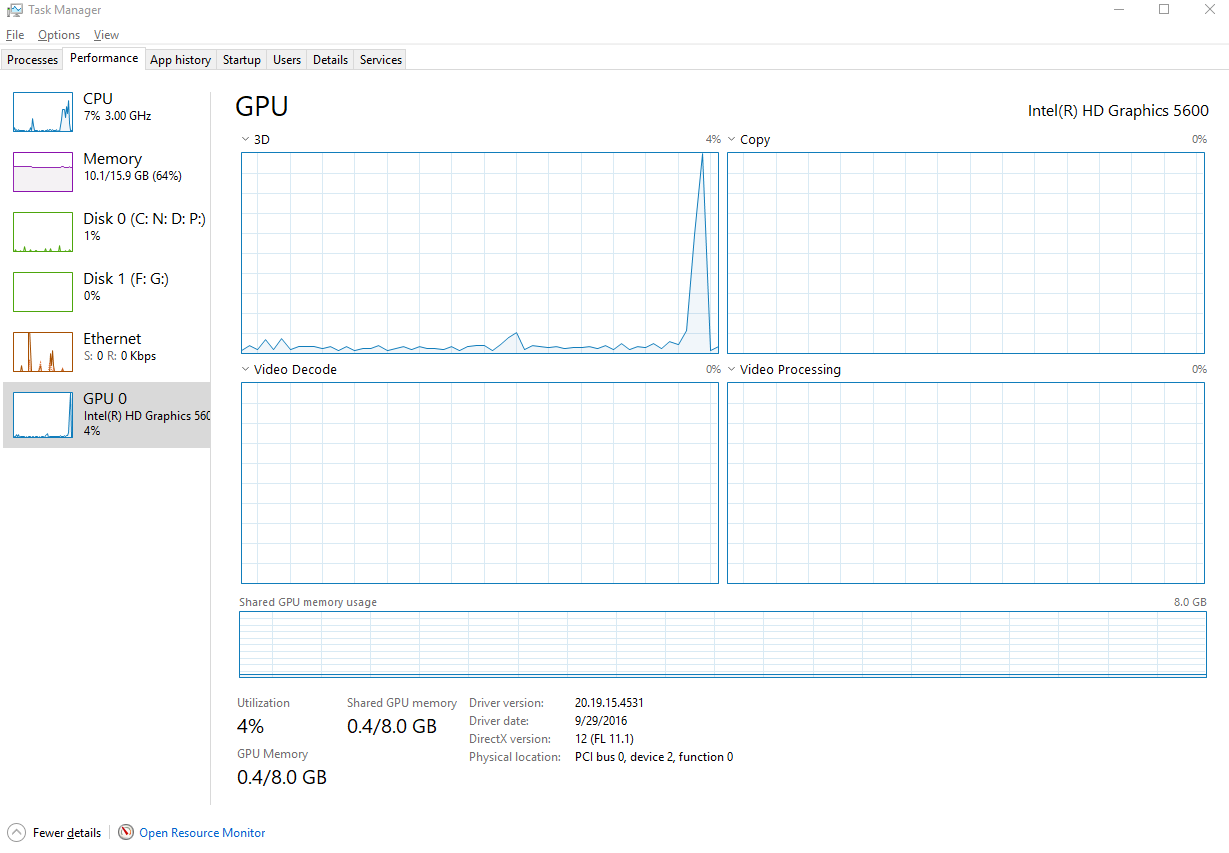## Finding prime numbers by executing code on CPU

Let's do the same thing but without using Aparapi.

```package com.logicbig.example;

import java.util.Arrays;
import java.util.stream.IntStream;

public class WithoutGpuExample {
public static void main(String[] args) {
final int size = 100000;
final int[] a = IntStream.range(2, size + 2).toArray();
final boolean[] primeNumbers = new boolean[size];

long startTime = System.currentTimeMillis();
for (int n = 0; n < size; n++) {
int num = a[n];
boolean prime = true;
for (int i = 2; i < num; i++) {
if (num % i == 0) {
prime = false;
//not using break for a fair comparision
}
}
}
System.out.printf("time taken: %s ms%n", System.currentTimeMillis() - startTime);
System.out.println(Arrays.toString(Arrays.copyOf(primeNumbers, 20)));//just print a sub array
}
}
```
`time taken: 15927 ms[true, true, false, true, false, true, false, false, false, true, false, true, false, false, false, true, false, true, false, false]`

As seen above, the CPU execution is way slower than the GPU execution time.

## Finding prime numbers by executing code in parallel on CPU

Let's do the same thing on CPU but in parallel by using Java 8 stream

```package com.logicbig.example;

import java.util.Arrays;
import java.util.stream.IntStream;

public class WithoutGpuParallelExample {
public static void main(String[] args) {
final int size = 100000;
final int[] a = IntStream.range(2, size + 2).toArray();

long startTime = System.currentTimeMillis();
Object[] primeNumbers = Arrays.stream(a)
.parallel()
.mapToObj(WithoutGpuParallelExample::isPrime)
.toArray();
System.out.printf("time taken: %s ms%n", System.currentTimeMillis() - startTime);
System.out.println(Arrays.toString(Arrays.copyOf(primeNumbers, 20)));//just print a sub array
}

private static boolean isPrime(int num) {
boolean prime = true;
for (int i = 2; i < num; i++) {
if (num % i == 0) {
prime = false;
//not using break for a fair comparision
}
}
return prime;
}
}
```
`time taken: 3238 ms[true, true, false, true, false, true, false, false, false, true, false, true, false, false, false, true, false, true, false, false]`

It's still slower than GPU execution.

## Setting execution mode with Kernel

Following method of `com.aparapi.Kernel` allows to set the execution mode explicitly:

`public void setExecutionModeWithoutFallback(EXECUTION_MODE executionMode)`

Where EXECUTION_MODE enum is defined as:

``` @Deprecated
public static enum EXECUTION_MODE {
AUTO,
NONE,
GPU,
CPU,
SEQ,
ACC;
....
}```

Even though `EXECUTION_MODE` has been deprecated, we can still experiment our first prime number example with CPU and JTP modes:

```public class ExecModeCpuExample {
public static void main(String[] args) {
final int size = 100000;
final int[] a = IntStream.range(2, size + 2).toArray();
final boolean[] primeNumbers = new boolean[size];

Kernel kernel = new Kernel() {
@Override
public void run() {
int gid = getGlobalId();
int num = a[gid];
boolean prime = true;
for (int i = 2; i < num; i++) {
if (num % i == 0) {
prime = false;
//break is not supported
}
}
}
};
long startTime = System.currentTimeMillis();
kernel.setExecutionModeWithoutFallback(Kernel.EXECUTION_MODE.CPU);
kernel.execute(size);
System.out.printf("time taken: %s ms%n", System.currentTimeMillis() - startTime);
System.out.println(Arrays.toString(Arrays.copyOf(primeNumbers, 20)));//just print a sub array
kernel.dispose();
}
}```
`time taken: 2368 ms[true, true, false, true, false, true, false, false, false, true, false, true, false, false, false, true, false, true, false, false]`

Still slower than the default GPU mode.

In upcoming tutorials we will go through more features of Aparapi.

## Example Project

Dependencies and Technologies Used:

• aparapi 1.10.0: A java OpenCl framework.
• JDK 1.8
• Maven 3.5.4

 Aparapi Framework Example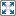Select All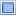Download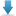• aparapi-getting-started-example
• src
• main
• java
• com
• logicbig
• example
• GpuExample.java
 Share